# RD Sharma Solutions for Class 9 Maths Chapter 11 Co-ordinate Geometry

## RD Sharma Solutions Class 9 Maths Chapter 11 – Free PDF Download

RD Sharma Class 9 Solutions Chapter 11 Coordinate Geometry is provided here. In this chapter, students will learn about coordinate geometry and concepts such as the cartesian plane, coordinates of a point, names and terms associated with the coordinate plane, notations, plotting points in the plane and more. Students can access RD Sharma Solutions from the links given below and use them while solving textbook problems. RD Sharma Class 9 Solutions contain solved exercises with explanations given by BYJU’S experts in a detailed manner.

Students can make use of the solutions updated for 2021-22 exams, to develop better Maths skills. The RD Sharma Solutions are free and students can view them online on the website or save it for later use. For effective exam preparation, students are recommended to solve all the questions of RD Sharma textbook following the answers formulated by expert teachers.

## Download PDF of RD Sharma Solutions for Class 9 Maths Chapter 11 Co-ordinate Geometry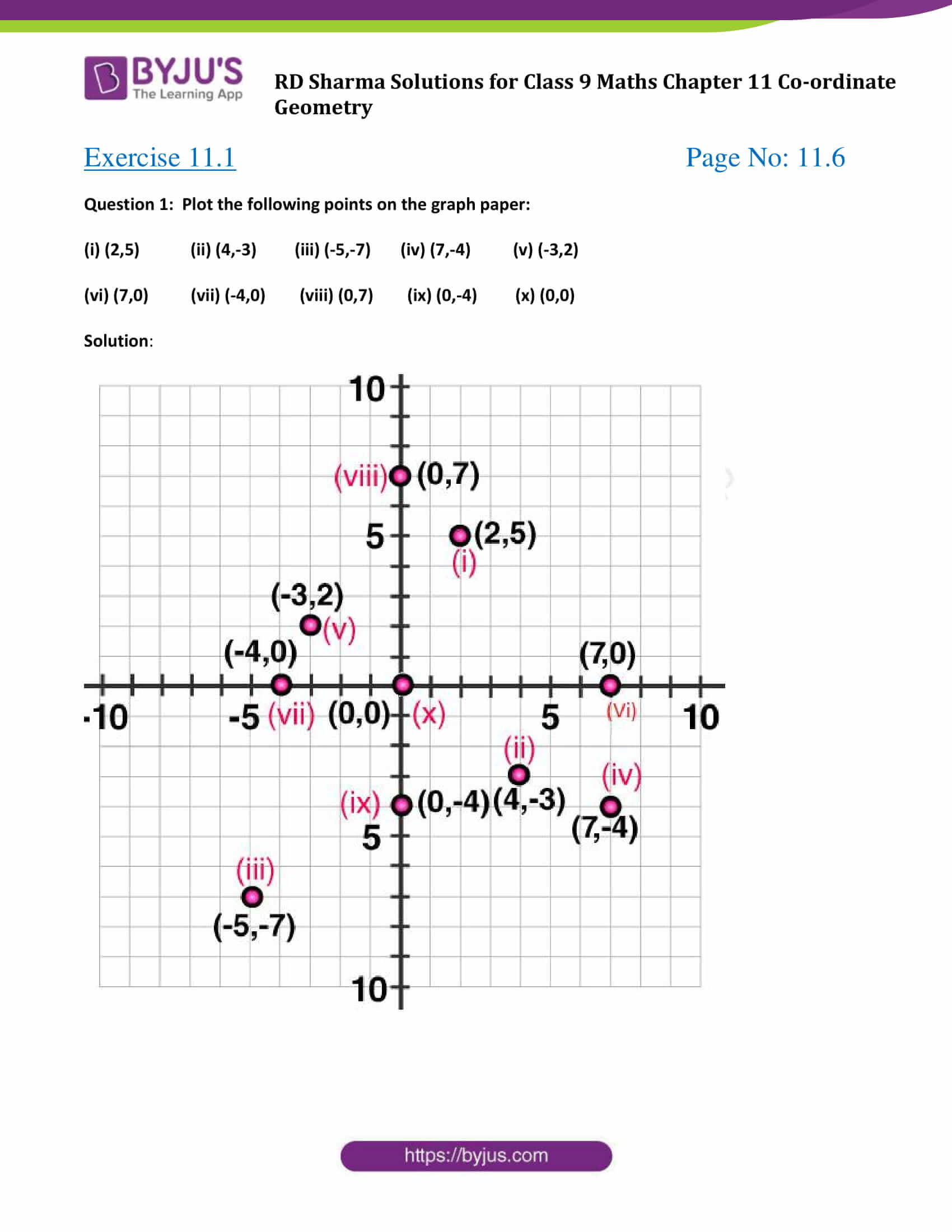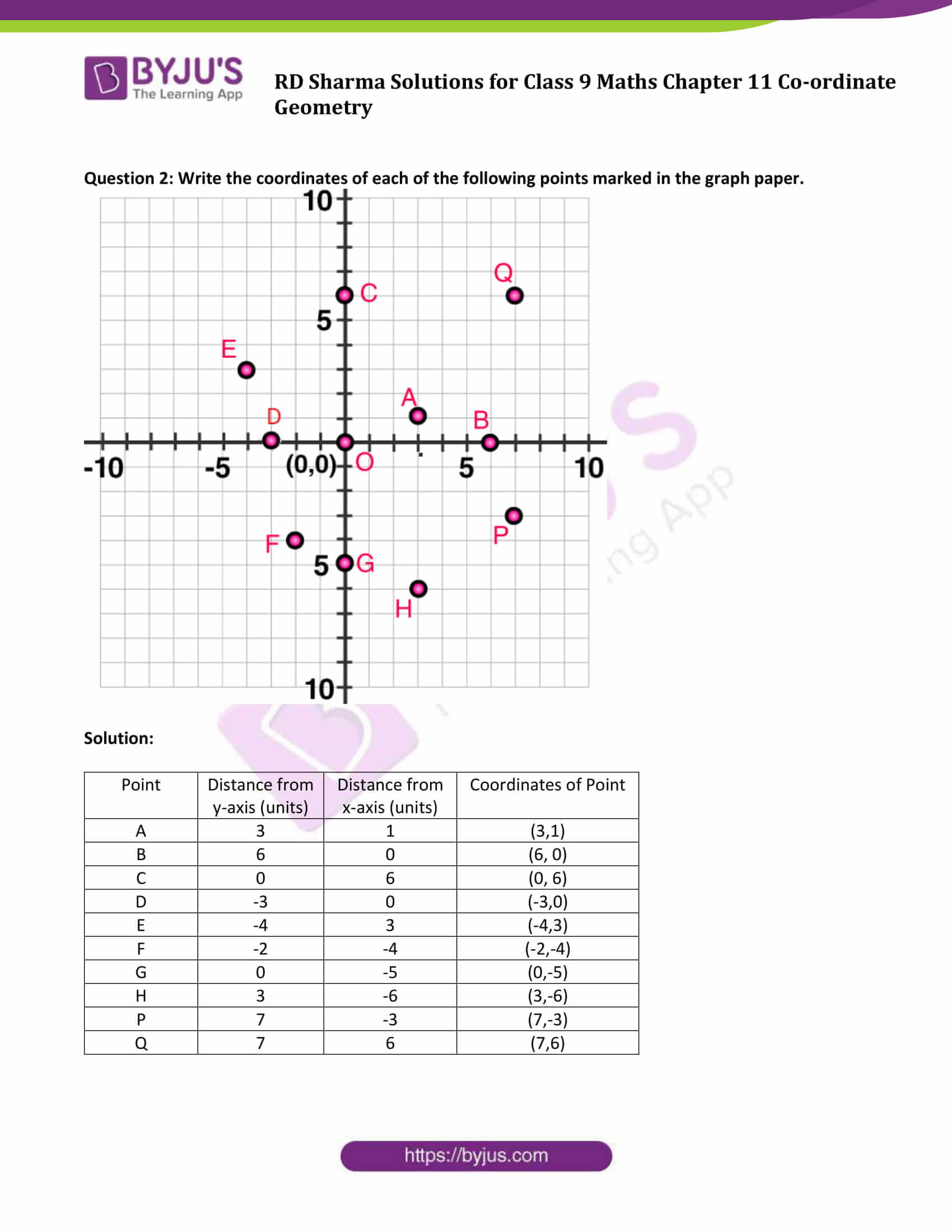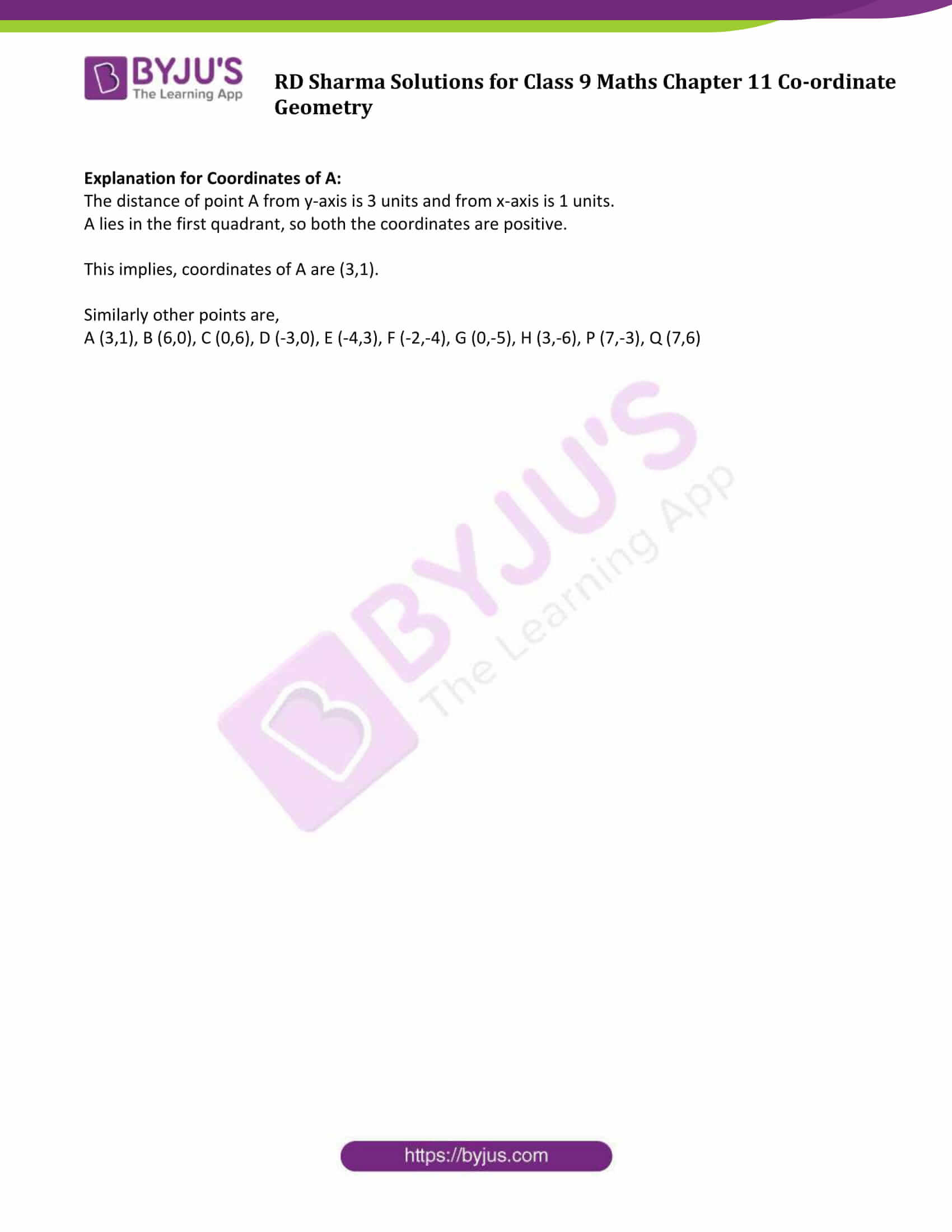### Access Answers to Maths RD Sharma Solutions for Class 9 Chapter 11 Co-ordinate Geometry

Exercise 11.1 Page No: 11.6

Question 1: Plot the following points on the graph paper:

(i) (2,5) (ii) (4,-3) (iii) (-5,-7) (iv) (7,-4) (v) (-3,2)

(vi) (7,0) (vii) (-4,0) (viii) (0,7) (ix) (0,-4) (x) (0,0)

Solution: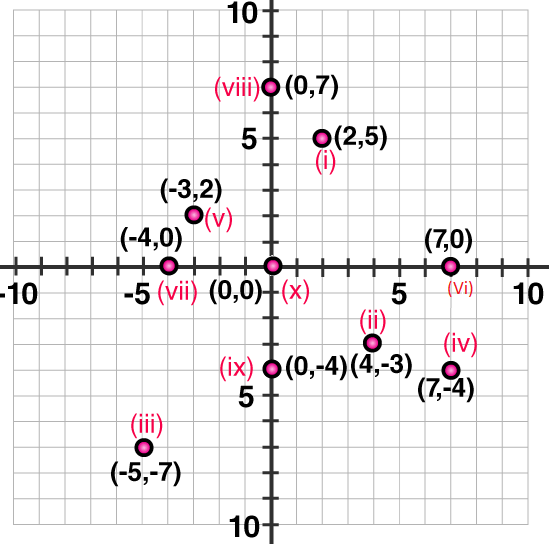Question 2: Write the coordinates of each of the following points marked in the graph paper.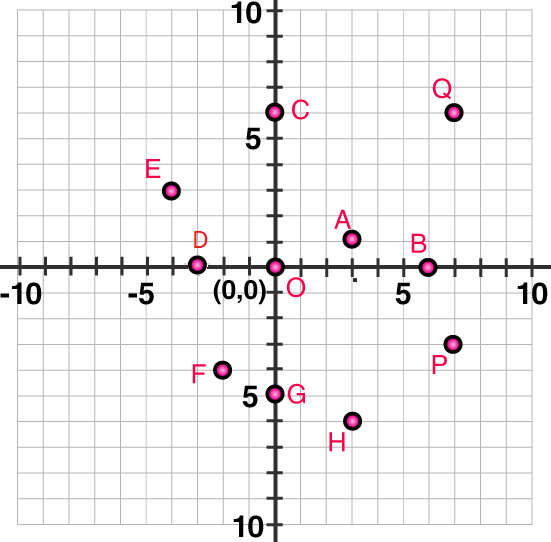Solution:

 Point Distance from y-axis (units) Distance from x-axis (units) Coordinates of Point A 3 1 (3,1) B 6 0 (6, 0) C 0 6 (0, 6) D -3 0 (-3,0) E -4 3 (-4,3) F -2 -4 (-2,-4) G 0 -5 (0,-5) H 3 -6 (3,-6) P 7 -3 (7,-3) Q 7 6 (7,6)

Explanation for Coordinates of A:

The distance of point A from y-axis is 3 units and from x-axis is 1 units.

A lies in the first quadrant, so both the coordinates are positive.

This implies, coordinates of A are (3,1).

Similarly other points are,

A (3,1), B (6,0), C (0,6), D (-3,0), E (-4,3), F (-2,-4), G (0,-5), H (3,-6), P (7,-3), Q (7,6)

### RD Sharma Solutions for Class 9 Maths Chapter 11 Co-ordinate Geometry

In the 11th Chapter of Class 9 RD Sharma Solutions students will study important concepts listed below:

• Rectangular or cartesian co-ordinates of a point
• Cartesian co-ordinates axes
• Plotting of points

Students can download and practice all chapters solutions enlisted in RD Sharma Class 9 textbook and score good marks.

## Frequently Asked Questions on RD Sharma Solutions for Class 9 Maths Chapter 11

### What are the main topics covered in RD Sharma Solutions for Class 9 Maths Chapter 11?

The main topics covered in RD Sharma Solutions for Class 9 Maths Chapter 11 are given below:
1. Rectangular or cartesian coordinates of a point
2. Cartesian coordinates axes